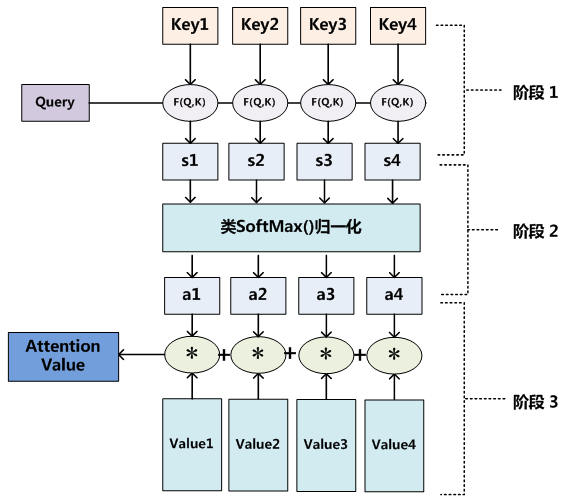# Attention机制的基本思想与实现原理

## 概述

Attention（注意力）机制如果浅层的理解，跟他的名字非常匹配。他的核心逻辑就是从关注全部到关注重点

### 研究进展

Attention机制最早在视觉领域提出，2014年Google Mind发表了《Recurrent Models of Visual Attention》，使Attention机制流行起来，这篇论文采用了RNN模型，并加入了Attention机制来进行图像的分类。

2015年，Bahdanau等人在论文《Neural Machine Translation by Jointly Learning to Align and Translate》中，将attention机制首次应用在nlp领域，其采用Seq2Seq+Attention模型来进行机器翻译，并且得到了效果的提升。

### 人类的视觉注意力

Attention 机制很像人类看图片的逻辑，当我们看一张图片的时候，我们并没有看清图片的全部内容，而是将注意力集中在了图片的焦点上。下图形象的展示了人类在看到一副图像时是如何高效分配有限的注意力资源的，其中红色区域表明视觉系统更关注的目标。很明显对于如图所示的场景，人们会把注意力更多的投入到人的脸部，文本的标题以及文章首句等位置。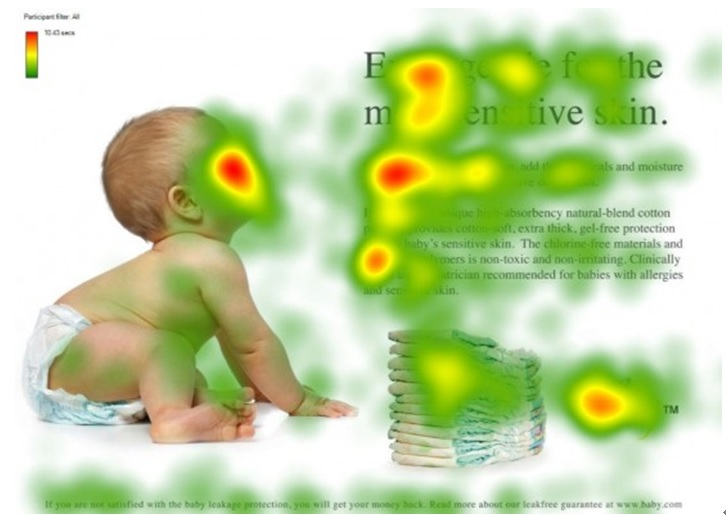## Encoder-Decoder的缺陷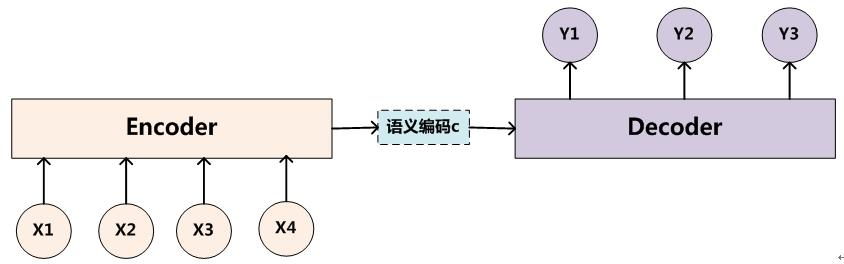$\begin{array}{l} \mathbf{Y}_{\mathbf{1}}=\mathbf{f} \mathbf{1}\left(\mathbf{C}\right) \\ \mathbf{Y}_{2}=\mathbf{f} \mathbf{1}\left(\mathbf{C}, \mathbf{y}_{\mathbf{1}}\right) \\ \mathbf{Y}_{3}=\mathbf{f} \mathbf{1}\left(\mathbf{C}, \mathbf{y}_{\mathbf{1}}, \mathbf{Y}_{2}\right) \end{array}$

• 不论输入和输出的长度是多少，中间的"语义编码C"长度都是固定的。
• 根据不同的任务可以选择不同的编码器和解码器（可以是一个RNN，但通常是其变种LSTM或者GRU）

## Attention机制

$(Tom,0.3) (Chase,0.2) (Jerry,0.5)$

### 语义编码的计算方法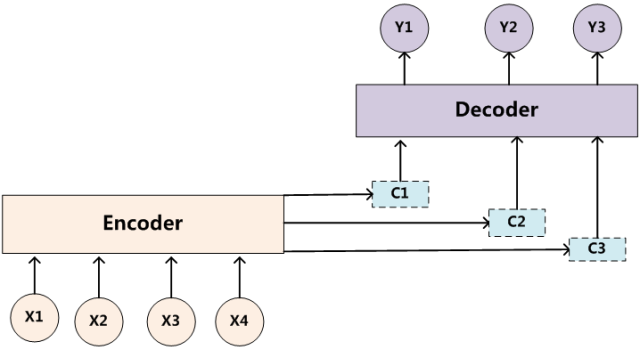$\begin{array}{l} \mathbf{Y}_{\mathbf{1}}=\mathbf{f} \mathbf{1}\left(\mathbf{C}_{\mathbf{1}}\right) \\ \mathbf{Y}_{2}=\mathbf{f} \mathbf{1}\left(\mathbf{C}_{2}, \mathbf{Y}_{\mathbf{1}}\right) \\ \mathbf{Y}_{3}=\mathbf{f} \mathbf{1}\left(\mathbf{C}_{3}, \mathbf{Y}_{\mathbf{1}}, \mathbf{Y}_{2}\right) \end{array}$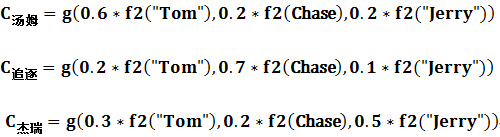$C_{i}=\sum_{j=1}^{L_{x}} a_{i j} h_{j}$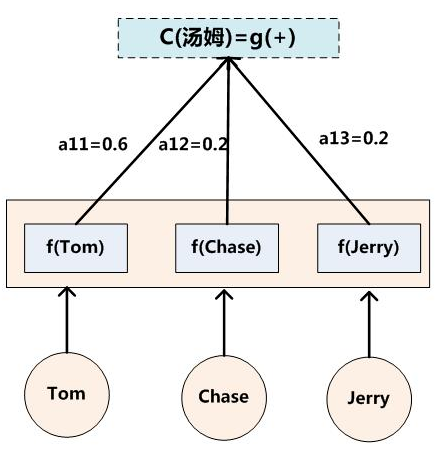### 注意力分配的方法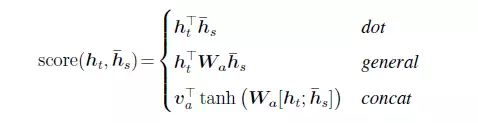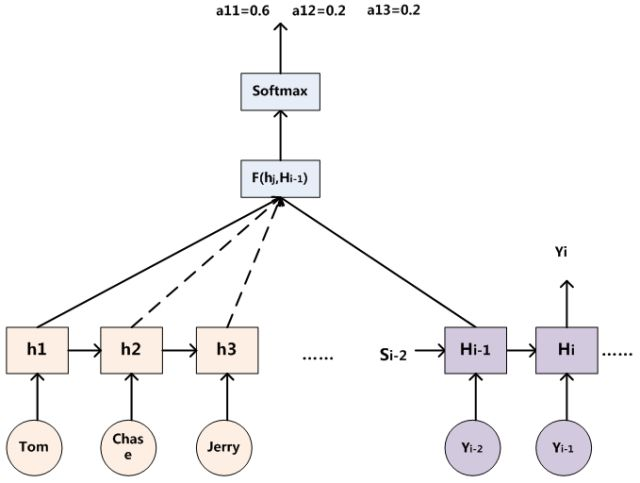Attention计算过程动图## Attention原理

Attention机制其实就是一系列注意力分配系数，也就是一系列权重参数罢了。

### 主流Attention框架

Attention是一组注意力分配系数，那么它是怎样实现的？这里要提出一个函数叫做attention函数，它是用来得到Attention value的。比较主流的attention框架如下：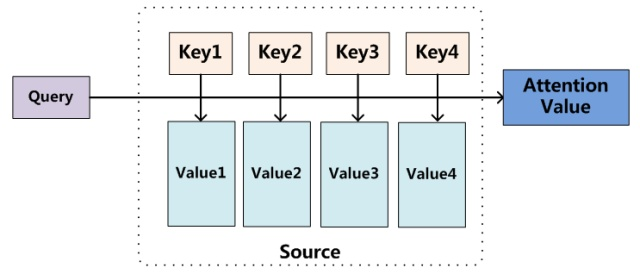### 三阶段计算Attention过程

Attention函数共有三步完成得到Attention值。

• 阶段1:Query与Key进行相似度计算得到权值
• 阶段2:对上一阶段的计算的权重进行归一化
• 阶段3:用归一化的权重与Value加权求和，得到Attention值